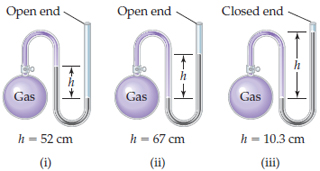# Problem: If the atmospheric pressure is 0.980 atm , what is the pressure of the enclosed gas in each of the three cases depicted in the drawing ?What is the pressure of the enclosed gas in (i)? Assume that the gray liquid is mercury.

###### FREE Expert Solution

A manometer allows you to determine the pressure of a gas (Pgas) sample by using the atmospheric pressure (Patm) and the difference in the height of mercury (h).

When Pgas is pushing harder than Patm###### Problem Details

If the atmospheric pressure is 0.980 atm , what is the pressure of the enclosed gas in each of the three cases depicted in the drawing?

What is the pressure of the enclosed gas in (i)? Assume that the gray liquid is mercury.